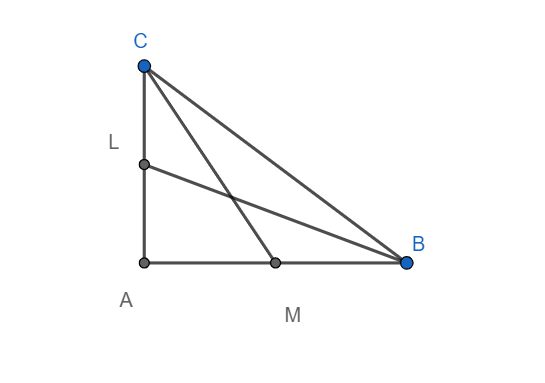QUESTION

# BL and BM are the medians of a right-angled triangle ABC, right-angled at A. Prove that $4\left( \text{B}{{\text{L}}^{2}}+\text{C}{{\text{M}}^{2}} \right)=5\text{B}{{\text{C}}^{2}}$

Hint: Use Pythagoras theorem in triangles AMC, ALB and ABC. Add the first two equations formed and using the third equation, simplify the expression and hence prove the result.

Pythagoras theorem: In a right-angled triangle the square of the length of the hypotenuse equals the sum of the squares of the lengths of the other two sides.In triangle AMC by Pythagoras theorem, we have
$\text{A}{{\text{M}}^{2}}+\text{A}{{\text{C}}^{2}}=\text{C}{{\text{M}}^{2}}\text{ (i)}$
Similarly in triangle ALB by Pythagoras theorem, we have
$\text{A}{{\text{L}}^{2}}+\text{A}{{\text{B}}^{2}}=\text{B}{{\text{L}}^{2}}\text{ (ii)}$
In triangle ABC, by Pythagoras theorem, we have
$\text{A}{{\text{B}}^{2}}+\text{A}{{\text{C}}^{2}}=\text{B}{{\text{C}}^{2}}\text{ (iii)}$
Adding equation (i) and equation (ii), we get
$\text{A}{{\text{M}}^{2}}+\text{A}{{\text{C}}^{2}}+\text{A}{{\text{L}}^{2}}+\text{A}{{\text{B}}^{2}}=\text{C}{{\text{M}}^{2}}+\text{B}{{\text{L}}^{2}}$
Since M is the midpoint of AB and L is the midpoint of AC, we have
$\text{AM=}\dfrac{\text{AB}}{2}$ and $\text{AL=}\dfrac{\text{AC}}{2}$
Hence, we have
\begin{align} & {{\left( \dfrac{\text{AB}}{2} \right)}^{2}}+{{\left( \dfrac{\text{AC}}{2} \right)}^{2}}+\text{A}{{\text{B}}^{2}}\text{+A}{{\text{C}}^{2}}=\text{B}{{\text{L}}^{2}}+\text{C}{{\text{M}}^{2}} \\ & \Rightarrow \dfrac{\text{A}{{\text{B}}^{2}}}{4}+\dfrac{\text{A}{{\text{C}}^{2}}}{4}+\text{A}{{\text{B}}^{2}}\text{+A}{{\text{C}}^{2}}=\text{B}{{\text{L}}^{2}}+\text{C}{{\text{M}}^{2}} \\ & \Rightarrow \dfrac{5}{4}\left( \text{A}{{\text{B}}^{2}}\text{+A}{{\text{C}}^{2}} \right)=\text{B}{{\text{L}}^{2}}+\text{C}{{\text{M}}^{2}} \\ \end{align}
Multiplying both sides by 4, we get
$5\left( \text{A}{{\text{B}}^{2}}\text{+A}{{\text{C}}^{2}} \right)=4\left( \text{B}{{\text{L}}^{2}}\text{+C}{{\text{M}}^{2}} \right)$
Now, from equation (iii), we have
$5\left( \text{B}{{\text{C}}^{2}} \right)=4\left( \text{B}{{\text{L}}^{2}}\text{+C}{{\text{M}}^{2}} \right)$
Hence, we have
$4\left( \text{B}{{\text{L}}^{2}}\text{+C}{{\text{M}}^{2}} \right)=5\text{B}{{\text{C}}^{2}}$
Hence proved.

Note: The above result can also be proved analytically i.e. using coordinate geometry.
Let $\text{A}\equiv \left( 0,0 \right),\text{B}\equiv \left( a,0 \right)$ and $\text{C}\equiv \left( 0,b \right)$be the coordinates of a right-angled triangle right angled at A.
Midpoint theorem: if $\text{A}\equiv \left( {{x}_{1}},{{y}_{1}} \right)$ and $\text{B}\equiv \left( {{x}_{2}},{{y}_{2}} \right)$, then the coordinates of the midpoint C of AB are given by $\text{C}\equiv \left( \dfrac{{{x}_{1}}+{{x}_{2}}}{2},\dfrac{{{y}_{1}}+{{y}_{2}}}{2} \right)$. We use the midpoint theorem on AC and AB.
Hence we have $\text{L}\equiv \left( 0,\dfrac{b}{2} \right)$ and $\text{M}\equiv \left( \dfrac{a}{2},0 \right)$
Hence $\text{BC=}\sqrt{{{a}^{2}}+{{b}^{2}}},\text{CM=}\sqrt{{{b}^{2}}+\dfrac{{{a}^{2}}}{4}}$ and $\text{BL=}\sqrt{{{a}^{2}}+\dfrac{{{b}^{2}}}{4}}$.
Hence we have
$\text{B}{{\text{L}}^{2}}+\text{C}{{\text{M}}^{2}}={{a}^{2}}+\dfrac{{{b}^{2}}}{4}+{{b}^{2}}+\dfrac{{{a}^{2}}}{4}=\dfrac{5}{4}\left( {{a}^{2}}+{{b}^{2}} \right)=\dfrac{5}{4}\text{B}{{\text{C}}^{2}}$
Multiplying both sides by 4 yields the result.
Hence proved.